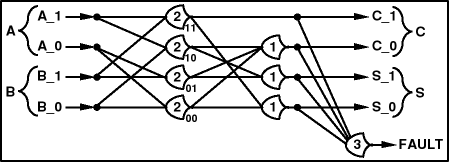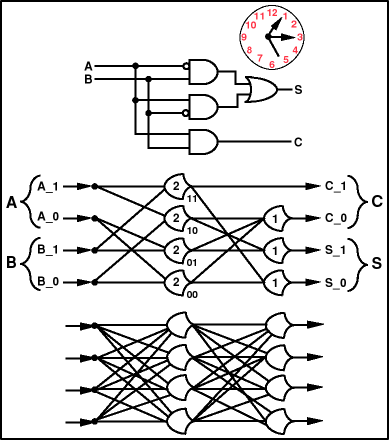Theseus Research : Technical Papers : NULL Convention Logic™ Page 14 of 26
Interesting Observations on Single Data Value NULL Convention Logic Circuits

A single data value NULL Convention Logic circuit can be conveniently monitored for faults in such a way that it cannot tell a lie as shown in Figure 17.Figure 17. Fault monitoring NULL Convention Logic circuit.

If exactly one DATA value is asserted in each output group it is the correct resolution of a complete input data set. If there are ever three DATA result values simultaneously asserted, it is an error and the threshold three gate will announce the error. If the circuit only asserts one DATA result value it will fail to announce completion of resolution and this can be detected with a watchdog timer. A NULL Convention Logic circuit will always either:

1. Assert a correct result.

2. Fail to complete resolution.

3. Assert an explicit error signal.

A fault will be detected as soon as it causes an actual resolution error. This holds for all single point faults. It is possible for a NULL Convention Logic circuit to tell a lie but it requires two coordinated faults that produce a valid encoding which is, nevertheless, erroneous.

Single data value NULL Convention Logic circuits are similar to neural nets as shown in Figure 18.Figure 18. Similarity to neural nets.

The single data value NULL Convention Logic circuit in the middle performs the same discrete symbolic processing as the Boolean circuit at the top, while at the same time being a more complete and autonomous expression of the process than the Boolean logic circuit. The fully connected neural net at the bottom, with an input layer, a hidden layer and an output layer can be configured to identically match the NULL Convention Logic circuit by setting the thresholds of each node and setting the weights of each connection appropriately to zero or one. The NULL Convention Logic circuit might be viewed as a pretrained neural net.